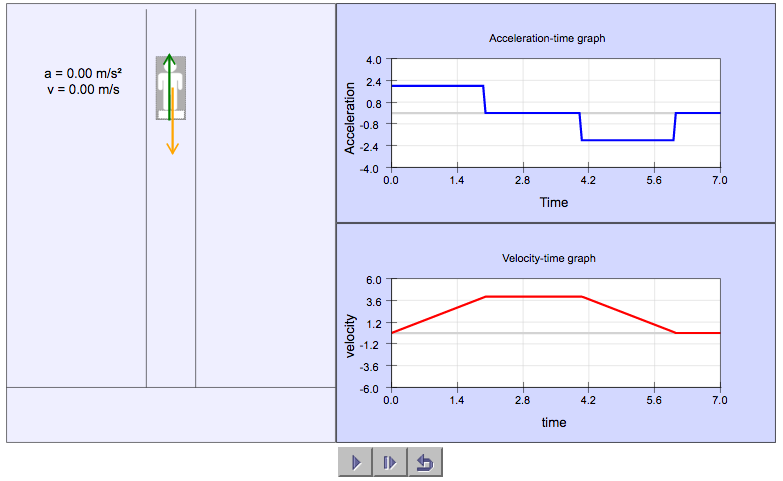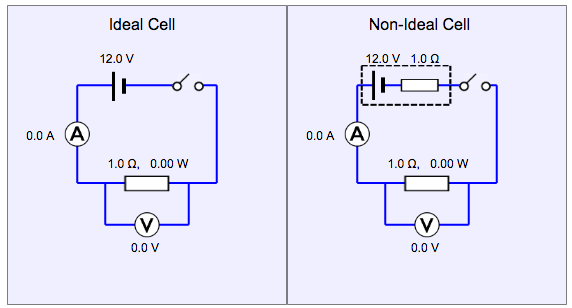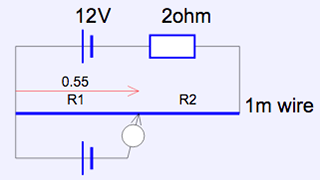# Month: November 2016

## Box on a Slope Simulation

This simulation allows students to observe the variation of the normal contact force N acting on a box placed on a frictionless slope with the angle of inclination of the slope changing. It also allows them to see how the Weight vector W can be resolved into two components, with the one perpendicular to the slope being equal at all times to N. Meanwhile, the component of W parallel to the slope being proportional and in the same direction as acceleration.## Man in Elevator SimulationIn this simulation, students can observe the variation of the normal contact force (N) and its effect on acceleration and velocity as an elevator moves upward.

Questions for students to work on can include:

1. Express the acceleration as a function of Normal Contact Force (N), Weight (W) and mass of the man.
2. Determine the distance travelled by the elevator.
3. Predict how the forces, acceleration and velocity will differ if the elevator was moving down instead.

## Internal Resistance and Maximum Power Theorem

I’ve created this simulation to demonstrate the effect of an internal resistance due to a cell on the potential difference and current of an external load.

One can also vary the internal resistance and external resistance to observe the maximum power theorem. The theorem states that for a given finite internal resistance, one can obtain the maximum external power only when the resistance of the load is equal to the internal resistance of the source.## Variable Resistor Simulation

My third simulation in as many days. This time round, I created some png files for electrical circuit components that can be used for future simulations. I also figured out how to add text in line with a variable in the drawing panel for the simulation, hence the units can now follow the values of the meter readings.## Simulation for Potentiometer

Continuing the course on Easy Java Simulation, I did this simple simulation on the Potentiometer, which allows students to first predict, and then verify the position of a jockey to achieve null deflection of a galvanometer.

Click on the image below to try it out and do give me your feedback. I’ll try to improve it with whatever little skill I have.I decided on a DC circuit simulation as I could not find many on Open Source Physics EJSS platform. With this as a template, I guess I can develop more simulations on the same topic when I have time.